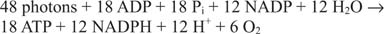## Energetics of Photosynthesis

This coupling is necessary because photosynthesis consumes a large amount of energy. To carry out the synthesis of a single molecule of glucose, the previous set of reactions must be carried out six times at a minimum.This requires the generation of six separate molecules of ribulose bisphosphate from ribulose phosphate, at the cost of one ATP each. Furthermore, two molecules of 1,3‐bisphosphoglycerate must be made from the two 3‐phosphoglycerates that are the initial product of each CO 2 fixation reaction. Conversion of each 1,3‐bisphosphoglycerate requires an NADPH as well; therefore, two NADPH equivalents are consumed for each CO 2 fixed. Another way of saying this is that carbon is reduced from an oxidation number of –4 in CO 2 to an oxidation number of zero in carbohydrate (CH 2O). Therefore, synthesis of one mole of glucose requires the input of 18 ATPs and 12 NADPHs.

Generating one NADPH from NADP requires the transfer of two electrons through PSI and PSII. Transfer of one electron requires that a photon be absorbed by both PSI and PSII, so that the generation of 12 ATPs requires the absorption of 12 × 2 × 2 = 48 photons. These 48 photons allow the synthesis of (just) 18 ATPs by coupling factor. Thus, the equation for photosynthesis can be written in two steps.

Light reactions:Dark reactions:The energy in 18 moles of photons of blue light is about 8000 kJ ( 1800 kcal) and the energy required to synthesize a mole of glucose from CO 2 and water is 2870 kJ (684 kcal). This means that the maximal efficiency of photosynthesis is about 35 percent. Roughly one third of the available energy from sunlight is converted into glucose under optimal conditions. This assumes that no cyclic electron flow occurs, which would further lower the efficiency of photosynthesis. Although 35 percent may seem inefficient, this value is actually quite good—about the same efficiency as a diesel engine.